# Difference between revisions of "Contragredient representation"

to a representationof a groupin a linear spaceThe representation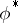of the same groupin the dual spaceofdefined by the rulefor all, wheredenotes taking adjoints.

More generally, ifis a linear space over the same fieldasand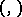is a non-degenerate bilinear form (pairing) on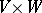with values in, then a representationofinis called the representation contragredient towith respect to the formif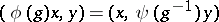for all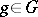,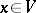,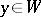.

For example, ifis the general linear group of a finite-dimensional space, then the natural representation ofin the space of covariant tensors of fixed rank onis the representation contragredient to the natural representation ofin the space of contravariant tensors of the same rank on.

Letbe finite-dimensional over, letbe a basis of it, and let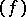be the basis dual to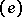in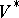. Then, for anyin, the matrix of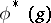in the basisis obtained from the matrix of the operator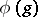in the basisby taking the transpose of the inverse. Ifis irreducible, then so is. Ifis a Lie group with Lie algebra, and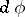andare the representations of the algebrainduced, respectively, by two representationsandofin spacesandthat are contragredient with respect to the pairing, then(*)

for all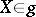,,. Representations of a Lie algebrasatisfying the condition (*) are also called contragredient representations with respect to.

Suppose further thatis a complex, connected, simply-connected semi-simple Lie group and thatis an irreducible finite-dimensional representation of it in a linear space. The weights of the representationare opposite to those of(see Weight of a representation of a Lie algebra), the lowest weight ofbeing opposite to the highest weight of(see Cartan theorem on the highest (weight) vector). The representationsandare equivalent if and only if there is a non-zero bilinear form onthat is invariant with respect to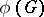. If such a form exists, then it is non-degenerate and either symmetric or skew-symmetric. The set of numerical marks of the highest weight of the representationis obtained from the set of numerical marks ofby applying the substitution induced by the following automorphismof the Dynkin diagram of simple rootsof:

a)takes each connected component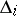,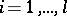, ofinto itself;

b) ifis a diagram of type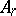,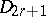or, then the restriction oftois uniquely defined as the unique element of order 2 in the automorphism group of; in the remaining cases the restriction oftois the identity.

How to Cite This Entry:
Contragredient representation. Encyclopedia of Mathematics. URL: http://encyclopediaofmath.org/index.php?title=Contragredient_representation&oldid=21833
This article was adapted from an original article by V.L. Popov (originator), which appeared in Encyclopedia of Mathematics - ISBN 1402006098. See original article